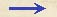Discorsi Propositions 3/01-th-01 Discorsi Proposition3/01-th-01{269} THEOREMA I, PROPOSITIO I. {269} THEOREM I, PROPOSITION I Proiectum, dum fertur motu composito ex horizontali aequabili et ex naturaliter accelerato deorsum, lineam semiparabolicam describit in sua latione. A projectile which is carried by a uniform horizontal motion compounded with a naturally accelerated vertical motion describes a path which is a semi-parabola.Intelligatur horizontales linea seu planum ab in sublimi positum, super quo ex a in b motu aequabili feratur mobile; deficiente vero plani fulcimento in b, superveniat ipsi mobili, a propria gravitate, motus naturalis deorsum iuxta perpendicularem bn.Intelligatur insuper plano ab in directum posita linea be, {30} tanquam temporibus effluxus seu mensura, super qua ad libitum notentur partes quotlibet temporis aequales, bc, ed, de; atque ex punctis b, c, d, e intelligantur productae lineae perpendiculo bn aequidistantes: in quarum prima accipiatur quaelibet pars ci; {273} cuius quadrupla sumatur in sequenti, df; nonupla, eh; et consequenter in reliquis secundum rationem quadratorum ipsarum cb, db, eb, seu dicamus in ratione earundem linearum duplicata.Quod si mobili ultra b versus c aequabili latione lato descensum perpendicularem secundum quantitatem ci superadditum intelligamus, reperietur tempore bc in termino i constitutum.Ulterius autem procedendo, tempore db, duplo scilicet bc, spatium descensus deorsum erit spatii primi ci quadruplum; demonstratum enim est in primo tractatu, spatia peracta a gravi, motu naturaliter accelerato, esse in duplicata ratione temporum: pariterque consequenter spatium eh, peractum tempore be, {10} erit ut 9: adeo ut manifeste constet, spatia eh, df, ci esse inter se ut quadrata linearum eb, db, cb.Ducantur modo a punctis i, f, h rectae io, fg, hl, ipsi eb aequidistantes: erunt hl, fg, io lineae lineis eb, db, cb, singulae singulis, aequales; nec non ipsae bo, bg, bl ipsis ci, df, eh aequales; eritque quadratum hl ad quadratum fg ut linea lb ad bg, et quadratum fg ad quadratum io ut gb ad bo; ergo puncta i f, h sunt in una eademque linea parabolica.Similiterque demonstrabitur, assumptis quibuscunque temporibus particulis aequalibus cuiuslibet magnitudinis, loca mobilis simili motu composito lati iisdem temporibus in eadem linea parabolica reperiri.Ergo patet propositum. Let us imagine an elevated horizontal line or plane ab along which a body moves with uniform speed from a to b. Suppose this plane to end abruptly at b; then at this point the body will, on account of its weight, acquire also a natural motion downwards along the perpendicular bn. Draw the line be along the plane ba (Condition 301D1) to represent the flow, or measure, of time; divide this line into a number of segments, bc, cd, de, representing equal intervals of time; from the points b, c, d, e, let fall lines which are parallel to the perpendicular bn. On the first of these lay off any distance ci, on the second a distance four times as long, df; on {273} the third, one nine times as long, eh; and so on, in proportion to the squares of cb, db, eb, or, we may say, in the squared ratio of these same lines. (Condition 301D1) Accordingly we see that while the body moves from b to c with uniform speed, it also falls perpendicularly through the distance ci, and at the end of the time-interval bc finds itself at the point i. (Condition 1/01-th-01) (Condition 2/02-th-02) In like manner at the end of the time-interval bd, which is the double of bc, the vertical fall will be four times the first distance ci; for it has been shown in a previous discussion that the distance traversed by a freely falling body varies as the square of the time; (Condition 1/01-th-01) (Condition 2/02-th-02)in like manner the space eh traversed during the time be will be nine times ci; thus it is evident that the distances eh, df, ci will be to one another as the squares of the lines be, bd, bc. Now from the points i, f, h draw the straight lines io, fg, hl parallel to be; these lines hl, fg, io are equal to eb, db and cb, respectively; so also are the lines bo, bg, bl respectively equal to ci, df, and eh. The square of hl is to that of fg as the line lb is to bg; and the square of fg is to that of io as gb is to bo; therefore the points i, f, h, lie on one and the same parabola. In like manner it may be shown that, if we take equal time-intervals of any size whatever, and if we imagine the particle to be carried by a similar compound motion, the positions of this particle, at the ends of these time-intervals, will lie on one and the same parabola. Q. E. D.Discorsi Propositions 3/01-th-01 Discorsi Proposition3/01-th-01